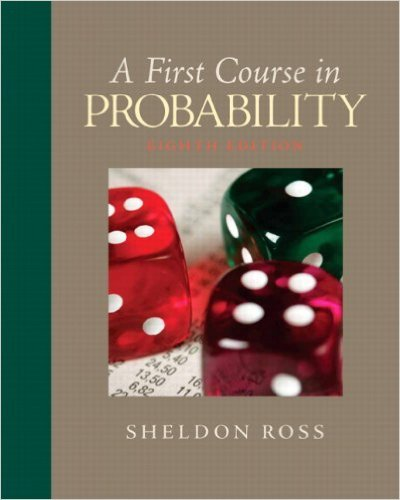×
Get Full Access to First Course In Probability - 8 Edition - Chapter 3 - Problem 3.50
Get Full Access to First Course In Probability - 8 Edition - Chapter 3 - Problem 3.50

×

# Suppose that an insurance company classifies peopleintoISBN: 9780136033134 163

## Solution for problem 3.50 Chapter 3

First Course in Probability | 8th Edition

• Textbook Solutions
• 2901 Step-by-step solutions solved by professors and subject experts
• Get 24/7 help from StudySoup virtual teaching assistantsFirst Course in Probability | 8th Edition

4 5 1 421 Reviews
24
4
Problem 3.50

Suppose that an insurance company classifies peopleinto one of three classes: good risks, averagerisks, and bad risks. The companys records indicatethat the probabilities that good-, average-, andbad-risk persons will be involved in an accidentover a 1-year span are, respectively, .05, .15, and.30. If 20 percent of the population is a good risk,50 percent an average risk, and 30 percent a badrisk, what proportion of people have accidents ina fixed year? If policyholder A had no accidentsin 1997, what is the probability that he or she is agood or average risk?

Step-by-Step Solution:
Step 1 of 3

9/29/2017 STT 351 STT 351 Notes 2 Given a random variable, we can associate probability to subsets of real numbers Probability Model Tossing a coin, Rolling a die, Roulette wheel The experiment has random outcomes. We want to assign chance (probability) to subsets outcomes. S = totality of outcomes A = a subcollection of outcomes Associate P(A) probability for A Example: Toss a fair coin S = {H,T} A = {H} P({H}) = ½ Two assumptions: 1. Number of outcomes is finite 2. Each outcome has equal chance P({H}) = ½ Example: Rolling a die S = {1,2,3,4,5,6} A = even number of outcomes = {2,4,6}

Step 2 of 3

Step 3 of 3

##### ISBN: 9780136033134

Unlock Textbook Solution